# Remove Element From List in R Language

• Last Updated : 21 Apr, 2021

R is an open-source programming language that is widely used as a statistical software and data analysis tool. R generally comes with the Command-line interface. R is available across widely used platforms like Windows, Linux, and macOS. Also, the R programming language is the latest cutting-edge tool. This article specifies how to remove one or more elements from a given list in R language. It will specify the number of elements to be removed from the list and print the rest elements. As a result, it will return a list of data after performing the operation. You will learn how to remove certain elements from the list.

### Method 1: Removing Item from the list using (-) method

Syntax: To remove elements using minus sign

my_list[- number]

Parameters:

number – Specifies the number of elements to be removed from the list

Return:

Return list after removing elements

Approach

• Create a vector.
• Remove one specific element using the (-) method.
• Delete multiple elements using the same method as illustrated in examples.

Example:

## R

 `# Create example list``my_list <- ``list``(a = ``c``(1, 4, 2, 7),                 ``                ``b = ``"geeksforgeeks"``,``                ``c = 2)``my_list`` ` `# remove elements ``my_list[- 1]`

Output: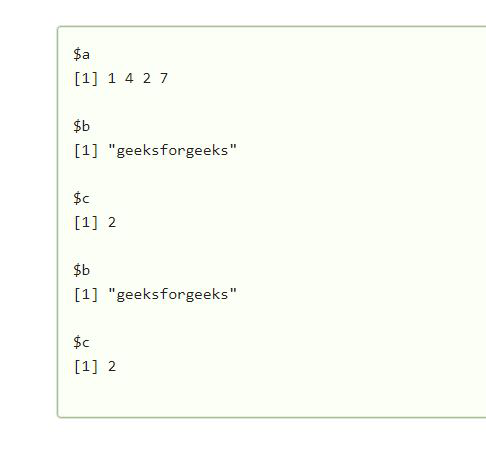Here, in the above example, my_list[- 1] will remove the data stored in the first list, and after removal of the first data list print the rest data on the screen.

### Method 2: Remove elements using NULL assignment

Syntax:

`my_list_2[number] <- NULL  `

Example:

## R

 `my_list <- ``list``(a = ``c``(1, 4, 2, 7),                 ``# Create example list``                ``b = ``"geeksforgeeks"``,``                ``c = 2)``my_list``my_list_2 <- my_list                               ``# Replicate list``my_list_2 <- ``NULL`                               `# Remove list elements with NULL``my_list_2`

Output :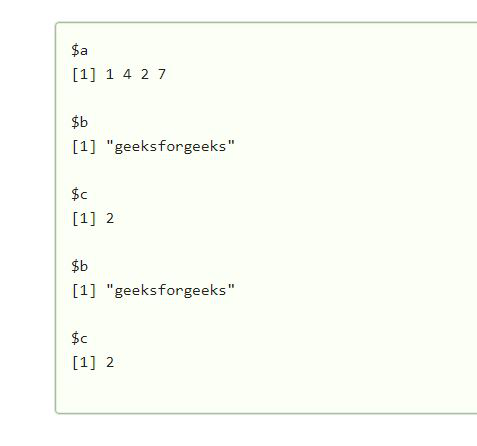The above example will remove elements by making them NULL in the list by using the my_list_2[] <- NULL   method. It will clear specific data and print the rest data on the screen.

### Method 3: Remove elements using the %in% operator

Syntax:

`my_list[names(my_list) %in% "number" == FALSE]    `

Example:

## R

 `# Create example list``my_list <- ``list``(a = ``c``(1, 4, 2, 7),                 ``                ``b = ``"geeksforgeeks"``,``                ``c = 2)``my_list`` ` `# Remove elements``my_list[``names``(my_list) %``in``% ``"c"` `== ``FALSE``]`

Output: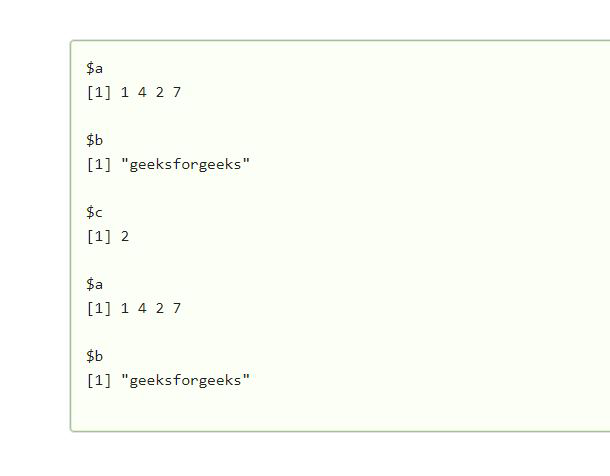my_list[names(my_list) %in% “c” == FALSE], with this method the list of data which are declared as false, which get removed from the data list and other will be printed on the screen.

### Method 4: Remove element using != operator

Syntax:

`my_list[names(my_list) != "number"]`

Example:

## R

 `# Create example list``my_list <- ``list``(a = ``c``(1, 4, 2, 7),                ``                ``b = ``"geeksforgeeks"``,``                ``c = 2)``my_list``my_list[``names``(my_list) != ``"a"``]`

Output: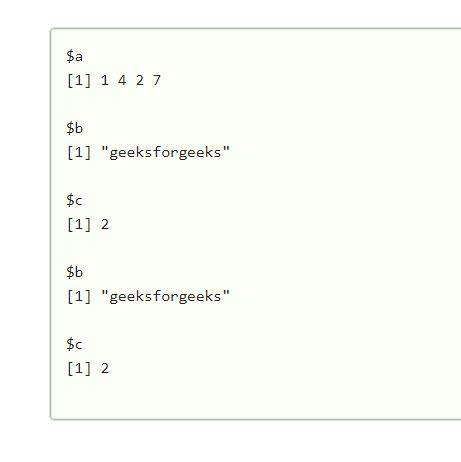my_list[names(my_list) != ” ”  In above code the data list number which is entered between double quote will get  removed .

Example:

## R

 `# Create example list``my_list <- ``list``(a = ``c``(1, 4, 2, 7),                 ``                ``b = ``"geeksforgeeks"``,``                ``c = 2)``my_list``my_list[- ``c``(1, 3)]`

Output: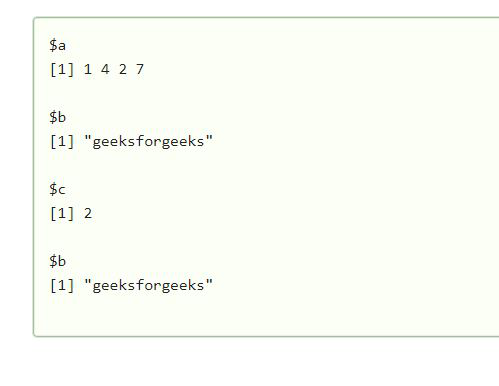My Personal Notes arrow_drop_up